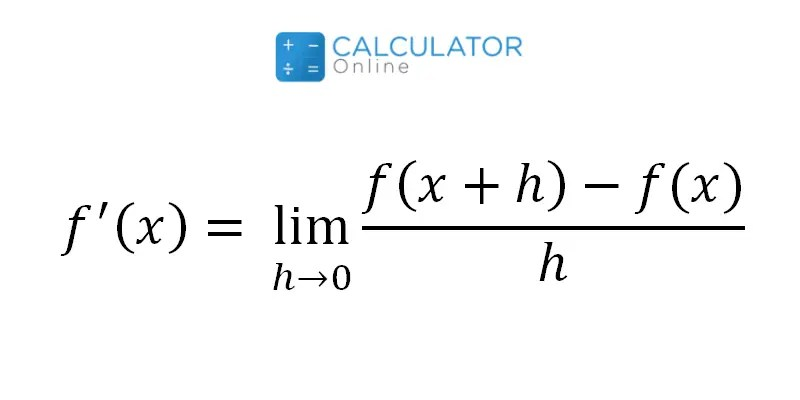# Differentiation Formula

Differentiation is a fundamental concept in calculus that deals with the rate at which a function changes. It is used in a wide range of fields, from physics to finance, to model and analyze complex phenomena. The process of differentiation involves finding the derivative of a function, which is the mathematical formula that describes its rate of change at any given point.

One of the most important aspects of differentiation is understanding the differentiation formula. The formula for differentiation is an essential tool for finding derivatives of functions and solving related problems. In this article, we will explore the differentiation formula, how it works, and some of its applications.

## The Differentiation Formula

The differentiation formula is a mathematical equation used to find the derivative of a function. It is based on the concept of limits, which is the idea of approaching a value from both sides to determine its value at that point. The formula is as follows:

f'(x) = lim(h→0) [f(x+h) – f(x)]/hwhere f'(x) represents the derivative of the function f(x) at the point x. The term (f(x+h) – f(x))/h represents the change in the function f(x) between x and x+h, divided by the distance h between the two points. The limit as h approaches zero ensures that we are taking the instantaneous rate of change of the function at the point x.

This formula is the basis for all differentiation techniques and is used to find derivatives of functions of any type. The power of the formula lies in its ability to calculate the rate of change of a function at any point, which is essential in many real-world applications.

## Applications of the Differentiation Formula

The differentiation formula has a wide range of applications in many fields. One of the most common applications is in physics, where it is used to model and analyze the motion of objects. The derivative of a position function gives us the velocity function, which describes the rate of change of position over time. Similarly, the second derivative gives us the acceleration function, which describes the rate of change of velocity over time.

In finance, the differentiation formula is used to calculate the risk and return of investments. The derivative of a profit function gives us the marginal profit function, which describes the rate of change of profit with respect to the number of units sold. This is essential for companies to determine their pricing strategy and optimize their profits.

In engineering, the differentiation formula is used to design and analyze complex systems. The derivative of a function describing a physical system gives us the sensitivity of the system to changes in its parameters. This is used to optimize the system’s performance and ensure that it meets the design requirements.

## Conclusion

The differentiation formula is a fundamental concept in calculus that is essential for understanding the rate of change of functions. It is based on the concept of limits and is used to find the derivative of a function at any point. The formula has a wide range of applications in physics, finance, engineering, and many other fields. Understanding the differentiation formula is crucial for anyone studying calculus or using it in their work.